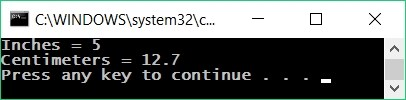# Reading Floating Point Numbers from the Console

To read a floating-point number (fractional number, non-integer) from the console use the following command:

var num = double.Parse(Console.ReadLine());


The above C# code first reads a text line from the console, then converts (parses) it to a floating-point number.

## Video: Reading Floating-Point Numbers

Watch a video lesson about how to read floating-point numbers: https://youtu.be/H2waLeIW70A.

## Example: Converting Inches into Centimeters

Let's write a program that reads a floating-point number in inches and converts it to centimeters:

Console.Write("Inches = ");
var inches = double.Parse(Console.ReadLine());
var centimeters = inches * 2.54;
Console.Write("Centimeters = ");
Console.WriteLine(centimeters);


Let's start the program and make sure that when a value in inches is entered, we obtain a correct output in centimeters:Note that if you enter and invalid number, e.g. "asfd", the program will crash with an error message (exception). We will learn how to handle exceptions later in the chapter "More Complex Loops".

### Testing in the Judge System

Test your solution here: https://judge.softuni.org/Contests/Practice/Index/504#1.